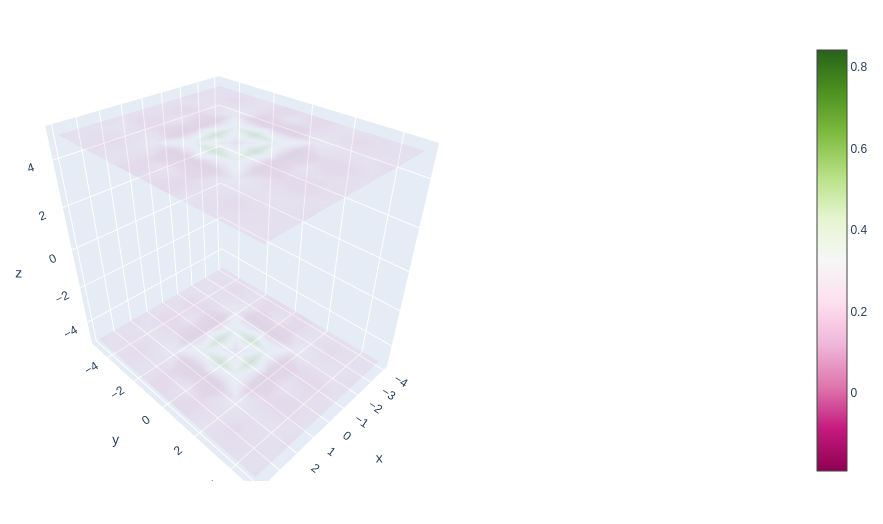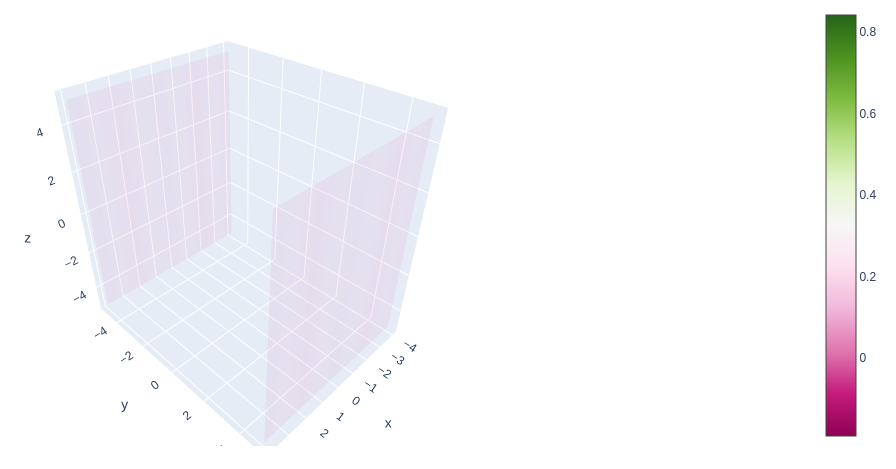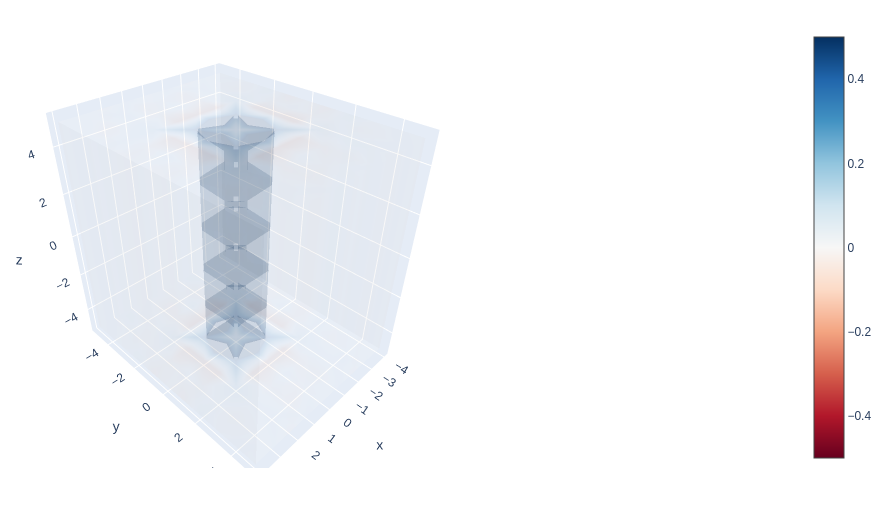Open in App
Not now

# 3D Volume Plots using Plotly in Python

• Last Updated : 10 Jul, 2020

Plotly is a Python library that is used to design graphs, especially interactive graphs. It can plot various graphs and charts like histogram, barplot, boxplot, spreadplot, and many more. It is mainly used in data analysis as well as financial analysis. Plotly is an interactive visualization library.

## Volume Plot in Plotly

A volume plot is a plot with go.volume which shows many partially transparent isosurfaces for rendering the volume. The opacityscale parameter of go. Volume results in a depth effect and generates better volume rendering. Three-dimensional volume visualization is a method that allows one to observe and manipulate 3D volumetric data. It represents 3D objects in terms of surfaces and edges approximated by polygons and lines.

Syntax: plotly.graph_objects.3d_Volume(arg=None, autocolorscale=None, caps=None, cauto=None, cmax=None, cmid=None, cmin=None, coloraxis=None, colorbar=None, colorscale=None, contour=None, customdata=None, customdatasrc=None, flatshading=None, hoverinfo=None, hoverinfosrc=None, hoverlabel=None, hovertemplate=None, hovertemplatesrc=None, hovertext=None, hovertextsrc=None, ids=None, idssrc=None, isomax=None, isomin=None, legendgroup=None, lighting=None, lightposition=None, meta=None, metasrc=None, name=None, opacity=None, opacityscale=None, reversescale=None, scene=None, showlegend=None, showscale=None, slices=None, spaceframe=None, stream=None, surface=None, text=None, textsrc=None, uid=None, uirevision=None, value=None, valuesrc=None, visible=None, x=None, xsrc=None, y=None, ysrc=None, z=None, zsrc=None, **kwargs)

Parameters:

x – Sets the X coordinates of the vertices on X axis.

y – Sets the Y coordinates of the vertices on Y axis.

z – Sets the Z coordinates of the vertices on Z axis.

value – Sets the 4th dimension (value) of the vertices.

Example:

## Python3

 `import` `plotly.graph_objects as go``import` `numpy as np`` ` ` ` `x1 ``=` `np.linspace(``-``4``, ``4``, ``9``) ``y1 ``=` `np.linspace(``-``5``, ``5``, ``11``) ``z1 ``=` `np.linspace(``-``5``, ``5``, ``11``) `` ` `X, Y, Z ``=` `np.meshgrid(x1, y1, z1)`` ` `values ``=` `(np.sin(X``*``*``2` `+` `Y``*``*``2``))``/``(X``*``*``2` `+` `Y``*``*``2``)`` ` `fig ``=` `go.Figure(data``=``go.Volume(``    ``x``=``X.flatten(),``    ``y``=``Y.flatten(),``    ``z``=``Z.flatten(),``    ``value``=``values.flatten(),``    ``opacity``=``0.1``,``    ``))`` ` `fig.show()`

Output:## Customizing caps in volume plot

In plotly, for more clear visualization of the internal surface, it is possible that the cap can be removed. Caps are visible by default.

Example 1:

## Python3

 `import` `plotly.graph_objects as go``import` `plotly.express as px``import` `numpy as np`` ` `df ``=` `px.data.tips()`` ` `x1 ``=` `np.linspace(``-``4``, ``4``, ``9``) ``y1 ``=` `np.linspace(``-``5``, ``5``, ``11``) ``z1 ``=` `np.linspace(``-``5``, ``5``, ``11``) `` ` `X, Y, Z ``=` `np.meshgrid(x1, y1, z1)`` ` `values ``=` `(np.sin(X``*``*``2` `+` `Y``*``*``2``))``/``(X``*``*``2` `+` `Y``*``*``2``)`` ` `fig ``=` `go.Figure(data``=``go.Volume(``    ``x``=``X.flatten(),``    ``y``=``Y.flatten(),``    ``z``=``Z.flatten(),``    ``value``=``values.flatten(),``    ``opacity``=``0.1``, ``    ``caps``=` `dict``(x_show``=``False``, y_show``=``False``, z_show``=``True``),``    ``))``fig.show()`

Output:Example 2:

## Python3

 `import` `plotly.graph_objects as go``import` `plotly.express as px``import` `numpy as np`` ` `df ``=` `px.data.tips()`` ` `x1 ``=` `np.linspace(``-``4``, ``4``, ``9``) ``y1 ``=` `np.linspace(``-``5``, ``5``, ``11``) ``z1 ``=` `np.linspace(``-``5``, ``5``, ``11``) `` ` `X, Y, Z ``=` `np.meshgrid(x1, y1, z1)`` ` `values ``=` `(np.sin(X``*``*``2` `+` `Y``*``*``2``))``/``(X``*``*``2` `+` `Y``*``*``2``)`` ` `fig ``=` `go.Figure(data``=``go.Volume(``    ``x``=``X.flatten(),``    ``y``=``Y.flatten(),``    ``z``=``Z.flatten(),``    ``value``=``values.flatten(),``    ``opacity``=``0.1``,``    ``caps``=` `dict``(x_show``=``False``, y_show``=``True``, z_show``=``False``),``    ``))``fig.show()`

Output:## Custom opacity scale

In plotly, it is possible to define the custom opacity scale, mapping scalar values proportionate to opacity values. The value is given from 0-1 and the maximum opacity is provided by the opacity keyword.

Example:

## Python3

 `import` `plotly.graph_objects as go``import` `plotly.express as px``import` `numpy as np`` ` `df ``=` `px.data.tips()`` ` `x1 ``=` `np.linspace(``-``4``, ``4``, ``9``) ``y1 ``=` `np.linspace(``-``5``, ``5``, ``11``) ``z1 ``=` `np.linspace(``-``5``, ``5``, ``11``) `` ` `X, Y, Z ``=` `np.meshgrid(x1, y1, z1)`` ` `values ``=` `(np.sin(X``*``*``2` `+` `Y``*``*``2``))``/``(X``*``*``2` `+` `Y``*``*``2``)`` ` `fig ``=` `go.Figure(data``=``go.Volume(``    ``x``=``X.flatten(),``    ``y``=``Y.flatten(),``    ``z``=``Z.flatten(), ``    ``isomin``=``-``0.5``,``    ``isomax``=``0.5``,``    ``value``=``values.flatten(),``    ``opacity``=``0.1``, ``    ``opacityscale``=``[[``-``0.5``, ``1``], [``-``0.2``, ``0``], [``0.2``, ``0``], [``0.5``, ``1``]],``    ``colorscale``=``'RdBu'``    ``))`` ` `fig.show()`

Output:My Personal Notes arrow_drop_up